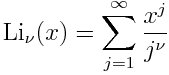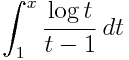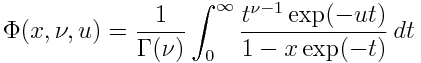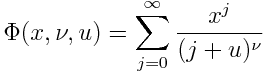# Dilogarithm, polylogarithm, and related functions

The functions dilogarithm, trilogarithm, and more generally polylogarithm are meant to be generalizations of the logarithm. I first came across the dilogarithm in college when I was evaluating some integral with Mathematica, and they’ve paid a visit occasionally ever since.

Unfortunately polylogarithms are defined in several slightly different and incompatible ways. I’ll start by following An Atlas of Functions and then mention differences in A&S, SciPy, and Mathematica.

## Polylogarithms

According to Atlas,

Polylogarithms are themselves special cases of Lerch’s function. Also known as Jonquière’s functions (Ernest Jean Philippe Fauque de Jonquières, 1820–1901, French naval officer and mathematician), they appear in the Feynman diagrams of particle physics.

The idea is to introduce an extra parameter ν in the power series for natural log:When ν = 1 we get the ordinary logarithm, i.e. polyln1 = log. Then polyln2 is the dilogarithm diln, and polyln3 is the trilogarithm triln.

One advantage of the definition in Atlas is that the logarithm is a special case of the polylogarithm. Other conventions don’t have this property.

## Other conventions

The venerable A&S defines dilogarithms in a way that’s equivalent to the negative of the definition above and does not define polylogarithms of any other order. SciPy’s special function library follows A&S. SciPy uses the name spence for the dilogarithm for reasons we’ll get to shortly.

Mathematica has the function PolyLog[ν, x] that evaluates toSo polylnν above corresponds to -PolyLog[ν, -x] in Mathematica. Matlab’s polylog is the same as Mathematica’s PolyLog.

## Relation to other functions

Spence’s integral is the function of x given by the integraland equals diln(x). Note that the SciPy function spence returns the negative of the integral above.

The Lerch function mentioned above is named for Mathias Lerch (1860–1922) and is defined by the integralThe connection with polylogarithms is easier to see from the series expansion:The connection with polylogarithms is thenNote that the Lerch function also generalizes the Hurwitz zeta function, which in turn generalizes the Riemann zeta function. When x = 1, the Lerch function reduces to ζ(ν, u).

Related: Applied complex analysis

## One thought on “Dilogarithm, polylogarithm, and related functions”

1. Don Fredkin

You might include among the sources of information the replacement for A&S, the “Digital Library of Mathematical Functions”, section 25.12, from NIST.